# 图像处理里面的匹配滤波

## 图像卷积与滤波的一些知识点

2015-10-12 21:24:06 zouxy09 阅读数 161905
• ###### OpenCV3.2 Java图像处理视频学习教程

OpenCV3.2 Java图像处理视频...主要内容包括opencv像素操作、滤波、边缘提取、直线与圆检测、形态学操作与分水岭、图像金子塔融合重建、多尺度模板匹配、opencv人脸检测、OpenCV跟Tomcat使用实现服务器端图像处理服务。

39课时 884分钟 4656人学习 贾志刚
免费试看

zouxy09@qq.com

http://blog.csdn.net/zouxy09

之前在学习CNN的时候，有对卷积进行一些学习和整理，后来就烂尾了，现在稍微整理下，先放上来，以提醒和交流。

线性滤波可以说是图像处理最基本的方法，它可以允许我们对图像进行处理，产生很多不同的效果。做法很简单。首先，我们有一个二维的滤波器矩阵（有个高大上的名字叫卷积核）和一个要处理的二维图像。然后，对于图像的每一个像素点，计算它的邻域像素和滤波器矩阵的对应元素的乘积，然后加起来，作为该像素位置的值。这样就完成了滤波过程。对图像和滤波矩阵进行逐个元素相乘再求和的操作就相当于将一个二维的函数移动到另一个二维函数的所有位置，这个操作就叫卷积或者协相关。卷积和协相关的差别是，卷积需要先对滤波矩阵进行180的翻转，但如果矩阵是对称的，那么两者就没有什么差别了。

Correlation 和 Convolution可以说是图像处理最基本的操作，但却非常有用。这两个操作有两个非常关键的特点：它们是线性的，而且具有平移不变性shift-invariant。平移不变性指我们在图像的每个位置都执行相同的操作。线性指这个操作是线性的，也就是我们用每个像素的邻域的线性组合来代替这个像素。这两个属性使得这个操作非常简单，因为线性操作是最简单的，然后在所有地方都做同样的操作就更简单了。

实际上，在信号处理领域，卷积有广泛的意义，而且有其严格的数学定义，但在这里不关注这个。

2D卷积需要4个嵌套循环4-double loop，所以它并不快，除非我们使用很小的卷积核。这里一般使用3x3或者5x5。而且，对于滤波器，也有一定的规则要求：

1）滤波器的大小应该是奇数，这样它才有一个中心，例如3x3，5x5或者7x7。有中心了，也有了半径的称呼，例如5x5大小的核的半径就是2。

2）滤波器矩阵所有的元素之和应该要等于1，这是为了保证滤波前后图像的亮度保持不变。当然了，这不是硬性要求了。

3）如果滤波器矩阵所有元素之和大于1，那么滤波后的图像就会比原图像更亮，反之，如果小于1，那么得到的图像就会变暗。如果和为0，图像不会变黑，但也会非常暗。

4）对于滤波后的结构，可能会出现负数或者大于255的数值。对这种情况，我们将他们直接截断到0和255之间即可。对于负数，也可以取绝对值。

上面说到，对图像的滤波处理就是对图像应用一个小小的卷积核，那这个小小的卷积核到底有哪些魔法，能让一个图像从惨不忍睹变得秀色可餐。下面我们一起来领略下一些简单但不简单的卷积核的魔法。

2.1、啥也不做

哈哈，大家可以看到啥了吗？这个滤波器啥也没有做，得到的图像和原图是一样的。因为只有中心点的值是1。邻域点的权值都是0，对滤波后的取值没有任何影响。下面我们动点真格的。

2.2、图像锐化滤波器Sharpness Filter

图像的锐化和边缘检测很像，首先找到边缘，然后把边缘加到原来的图像上面，这样就强化了图像的边缘，使图像看起来更加锐利了。这两者操作统一起来就是锐化滤波器了，也就是在边缘检测滤波器的基础上，再在中心的位置加1，这样滤波后的图像就会和原始的图像具有同样的亮度了，但是会更加锐利。我们把核加大，就可以得到更加精细的锐化效果另外，下面的滤波器会更强调边缘：主要是强调图像的细节。最简单的3x3的锐化滤波器如下：实际上是计算当前点和周围点的差别，然后将这个差别加到原来的位置上。另外，中间点的权值要比所有的权值和大于1，意味着这个像素要保持原来的值。

2.3、边缘检测Edge Detection

我们要找水平的边缘：需要注意的是，这里矩阵的元素和是0，所以滤波后的图像会很暗，只有边缘的地方是有亮度的。为什么这个滤波器可以寻找到水平边缘呢？因为用这个滤波器卷积相当于求导的离散版本：你将当前的像素值减去前一个像素值，这样你就可以得到这个函数在这两个位置的差别或者斜率。下面的滤波器可以找到垂直方向的边缘，这里像素上和下的像素值都使用：再下面这个滤波器可以找到45度的边缘：取-2不为了什么，只是为了让矩阵的元素和为0而已。那下面这个滤波器就可以检测所有方向的边缘:为了检测边缘，我们需要在图像对应的方向计算梯度。用下面的卷积核来卷积图像，就可以了。但在实际中，这种简单的方法会把噪声也放大了。另外，需要注意的是，矩阵所有的值加起来要是0.2.4、浮雕Embossing Filter

浮雕滤波器可以给图像一种3D阴影的效果。只要将中心一边的像素减去另一边的像素就可以了。这时候，像素值有可能是负数，我们将负数当成阴影，将正数当成光，然后我们对结果图像加上128的偏移。这时候，图像大部分就变成灰色了。

下面是45度的浮雕滤波器我们只要加大滤波器，就可以得到更加夸张的效果了这种效果非常的漂亮，就像是将一副图像雕刻在一块石头上面一样，然后从一个方向照亮它。它和前面的滤波器不同，它是非对称的。另外，它会产生负数值，所以我们需要将结果偏移，以得到图像灰度的范围。A：原图像。B：锐化。C：边缘检测。D：浮雕

2.5、均值模糊Box Filter (Averaging)

我们可以将当前像素和它的四邻域的像素一起取平均，然后再除以5，或者直接在滤波器的5个地方取0.2的值即可，如下图：可以看到，这个模糊还是比较温柔的，我们可以把滤波器变大，这样就会变得粗暴了：注意要将和再除以13.所以，如果你想要更模糊的效果，加大滤波器的大小即可。或者对图像应用多次模糊也可以。2.6、高斯模糊

均值模糊很简单，但不是很平滑。高斯模糊就有这个优点，所以被广泛用在图像降噪上。特别是在边缘检测之前，都会用来移除细节。高斯滤波器是一个低通滤波器。2.7、运动模糊Motion Blur

运动模糊可以通过只在一个方向模糊达到，例如下面9x9的运动模糊滤波器。注意，求和结果要除以9。这个效果就好像，摄像机是从左上角移动的右下角。

对图像处理而言，存在两大类的方法：空域处理和频域处理！空域处理是指直接对原始的像素空间进行计算，频率处理是指先对图像变换到频域，再做滤波等处理。

3.1、空域计算-直接2D卷积

3.1.1、2D卷积

直接2D卷积就是一开始说的那样，对于图像的每一个像素点，计算它的邻域像素和滤波器矩阵的对应元素的乘积，然后加起来，作为该像素位置的值。直接的实现也称为暴力实现brute force，因为它严格按照定义来实现，没有任何优化。当然了，在并行实现里面，它也是比较灵活的。另外，也存在一个优化版本，如果我们的kernel是separable可分的，那么就可以得到一个快5倍左右的卷积方法。

2.1.2、边界处理

那卷积核遇到图像边缘怎么办？例如图像顶部的像素，它的上面已经没有像素了，那么它的值如何计算？目前有四种主流的处理方法，我们用一维卷积和均值滤波来说明下。

我们在1D图像中，用每个像素和它的二邻域的平均值来取代它的值。假设我们有个1D的图像I是这样的：对非图像边界的像素的操作比较简单。假设我们对I的第四个像素3做局部平均。也就是我们用2,3和7做平均，来取代这个位置的像素值。也就是，平均会产生一副新的图像J，这个图像在相同位置J (4) = (I(3)+I(4)+I(5))/3 = (2+3+7)/3 = 4。同样，我们可以得到J(3) = (I(2)+I(3)+I(4))/3 =(4+2+3)/3 = 3。需要注意的是，新图像的每个像素都取决于旧的图像，在计算J (4)的时候用J (3)是不对的，而是用I(3)，I(4)和I(5)。所以每个像素都是它和它邻域两个像素的平均。平均是线性的操作，因为每个新的像素都是旧像素的线性组合。对卷积，也有必须要考虑的情况是，在图像边界的时候，怎么办？J(1)的值应该是什么？它取决于I(0)，I(1)和I(2)。但是我们没有I(0)呀！图像左边没有值了。有四种方式来处理这个问题：

1）第一种就是想象I是无限长的图像的一部分，除了我们给定值的部分，其他部分的像素值都是0。在这种情况下，I(0)=0。所以J(1) = (I(0) + I(1) + I(2))/3 = (0 + 5 + 4)/3= 3. 同样，J(10) = (I(9)+I(10)+I(11))/3 = (3+ 6 + 0)/3 = 3.2）第二种方法也是想象I是无限图像的一部分。但没有指定的部分是用图像边界的值进行拓展。在我们的例子中，因为图像I最左边的值I(1)=5，所以它左边的所有值，我们都认为是5 。而图像右边的所有的值，我们都认为和右边界的值I(10)一样，都是6。这时候J(1) = (I(0) + I(1) + I(2))/3 = (5 + 5 + 4)/3= 14/3. 而J(10) = (I(9)+I(10)+I(11))/3 = (3 + 6 + 6)/3 = 5。3）第三种情况就是认为图像是周期性的。也就是I不断的重复。周期就是I的长度。在我们这里，I(0)和I(10)的值就是一样的，I(11)的值和I(1)的值也是一样的。所以J(1) = (I(0) + I(1) + I(2))/3= (I(10) + I(1)+ I(2))/3 = (6 + 5 + 4)/3 = 5 。4）最后一种情况就是不管其他地方了。我们觉得I之外的情况是没有定义的，所以没办法使用这些没有定义的值，所以要使用图像I没有定义的值的像素都没办法计算。在这里，J(1)和J(10)都没办法计算，所以输出J会比原图像I要小。

这四种方法有各自的优缺点。如果我们想象我们使用的图像只是世界的一个小窗口，然后我们需要使用窗口边界外的值，那么一般来说，外面的值和边界上的值是几乎相似的，所以第二种方法可能更说得过去。

2.2、频域计算-快速傅里叶变换FFT卷积

这个快速实现得益于卷积定理：时域上的卷积等于频域上的乘积。所以将我们的图像和滤波器通过算法变换到频域后，直接将他们相乘，然后再变换回时域（也就是图像的空域）就可以了。o表示矩阵逐元素相乘。那用什么方法将空域的图像和滤波器变换到频域了。那就是鼎鼎大名的Fast Fourier Transformation 快速傅里叶变换FFT（其实，在CUDA里面，已经实现了FFT了）。

要在频域中对一副图像进行滤波，滤波器的大小和图像的大小必须要匹配，这样两者的相乘才容易。因为一般滤波器的大小比图像要小，所以我们需要拓展我们的kernel，让它和图像的大小一致。因为CUDA中的FFT实现是周期的，所以kernel的值也要安排成这样，以支持这种周期性。

为了保证图像边界的像素也可以得到响应输出，我们也需要拓展我们的输入图像。同时，拓展的方式也要支持周期表达。如果只是使用卷积定理，没有对输入进行任何修改的话，那么我们得到的是周期卷积的结果。但这可能不是我们要的，因为周期卷积会对输入数据进行周期填补，引入一些artifacts。四、实验代码

这是第二部分的Matlab实验代码：

clear,close all, clc

%% definefilter
% -----Identity filter -----
kernel =[0, 0, 0
0, 1, 0
0, 0, 0];

% -----Average Blur -----
kernel =[0, 1, 0
1, 1, 1
0, 1, 0] / 5;

% -----Gaussian Blur -----
kernel =fspecial('gaussian', 5 , 0.8);

% -----Motion Blur -----
kernel =[1, 0, 0, 0, 0
0, 1, 0, 0, 0
0, 0, 1, 0, 0
0, 0, 0, 1, 0
0, 0, 0, 0, 1] / 5;

% -----Edges Detection -----
kernel =[-1, -1, -1
-1, 8, -1
-1, -1, -1];

% -----Sharpen filter -----
kernel =[-1, -1, -1
-1, 9, -1
-1, -1, -1];

% -----Emboss filter -----
kernel =[-1, -1, 0
-1,  0,1
0,   1,1];

%% convolethe image with defined kernel or filter
result =zeros(size(image));
result(:,:, 1) = conv2(double(image(:, :, 1)), double(kernel), 'same');
result(:,:, 2) = conv2(double(image(:, :, 2)), double(kernel), 'same');
result(:,:, 3) = conv2(double(image(:, :, 3)), double(kernel), 'same');

%% showthe result
imshow(image);
figure
imshow(uint8(result))

 Correlation and Convolution.pdf

## [数字图像处理]空间滤波

2013-10-20 17:28:04 thnh169 阅读数 15357
• ###### OpenCV3.2 Java图像处理视频学习教程

OpenCV3.2 Java图像处理视频...主要内容包括opencv像素操作、滤波、边缘提取、直线与圆检测、形态学操作与分水岭、图像金子塔融合重建、多尺度模板匹配、opencv人脸检测、OpenCV跟Tomcat使用实现服务器端图像处理服务。

39课时 884分钟 4656人学习 贾志刚
免费试看

## 1.空间滤波

空间滤波，就是直接在灰度值上，做一些滤波操作。滤波一词，其实来源于频域，将某个频率成分滤除的意思。大部分线性的空间滤波器（比如均值滤波器），是在空间上进行一些灰度值上的操作，这个线性空间滤波器与频域滤波器有一一对应的关系（比如均值滤波器其本质就是低通滤波器），这样会有助于理解这个滤波器的特性。然而，对于非线性的滤波器（比如最大值，最小值和中央值滤波器）的话，则没有这样一个一一对应的关系。

线性空间滤波所使用的运算是卷积，其计算如下所示。这个式子可能会出现两个问题。这次是在空间域进行操作的，所以上式应该没什么问题。但是，如果换到频域，我们会发现，我们使用的滤波器是非因果的，按照我之前在数字信号处理的那一节，非因果具有零相位特性，但是是不可实现的，因为需要未来的输入。而在这，我们在图像处理的时候，都是一帧一帧的处理，所以，这里非因果性不是问题。而最重要的是，零相位特性不会使得图像变形，这是很重要的。

还有一个问题就是边界问题，当滤波器的中心靠近图像边缘时候，滤波器的一部分会位于图像外，那么此时，我们通常会采用填0的操作来解决。但是，一些场合，直接填0操作会使得操作后的图像出现黑边。所以，常用的操作还有，①选择距离最近的点填充，②填充的点为圆图像的镜像，③将原图像当做周期信号来填充。

## 2.几个典型的空间滤波器

### 2.1平滑滤波器

在空间域上考虑，我们所指的平滑滤波器，有平均滤波与加权平均滤波两种形式。我们将其放到频域去考虑的话，其实这是一个很典型的低通滤波器。这个滤波器会滤掉高频成分，所以可以使得图像平滑。其频率响应如下所示。3X3平均滤波的频率响应3X3加权平均滤波的频率响应

首先，对于两个滤波器的振幅特性。平均滤波器的通带要比加权平均滤波器的窄，故使用平均滤波器处理的图像要比加权滤波器处理的图像要模糊一些。

注意平均滤波器的相位特性，其相位特性并不是一个平面，有的地方的值为π。首先，平均滤波器是一个偶实函数，其频率响应是一个实函数。但是，其频率响应有的部分为负值，这就造成了Matlab的angle()的计算结果为π。其实其还是具有0相位特性的。

用其处理实际处理图像的话，会得到以下结果。看其处理结果，其实很难分辨出有什么区别。所以，加权平均滤波器和平均滤波器的区别，从频率响应来看的话，容易明白一些。本文只是简单的介绍一下均值滤波器，详细的，请参看[数字图像处理]图像去噪初步(1)--均值滤波器

### 2.2统计排序滤波器

统计排序滤波器的运用也广泛，其是很典型的非线性滤波器。主要包括了，最大值滤波器，最小值滤波器，中央值滤波器等等。这里作为代表的，主要说中央值滤波器，中央值滤波对于去除椒盐噪声特别有效。

所谓中央值滤波器，就是将滤波器范围内的像素的灰度值，进行排序，选出中央值作为这个像素的灰度值。同理可解释最大值滤波器与最小值滤波器。

我们将一幅图像添加椒盐噪声，然后尝试着用中央值滤波器去除。### 2.3锐化滤波器

使用平均滤波器，可以将图像平滑，其本质是将图像在滤波器范围内求平均值。从频域上来看，平均滤波器是低通滤波器。然而，所谓的锐化，即是将图像的细节强调出来。这里进行了一个假设，假设细节部分是图像高频成分。从这里看来，其实锐化滤波器是与平均滤波器是相反的操作。

对于一个一次元函数，其一阶微分为其频率响应如下所示。我们可以看出，八方向的拉普拉斯滤波器对于高频成分的强调效果较强。其低频部分最小值为0，这意味着，进行拉普拉斯滤波之后，其实只剩下图像的高频部分了（在空间域里来讲，只剩下边缘部分了）。所以，若用于图像锐化的话，可以将所得结果叠加至原图像，其实也就相当与滤波器的振幅特性往上移动1，保证低频部分不变，强调高频部分。### 2.4高提升滤波

高提升滤波一般用于使得图片更加清晰。其步骤大致如下，首先将图片模糊化，然后从原图中，将其模糊形式去除。其实，高提升滤波也是一种锐化滤波，其强调的也是图像的边缘部分（或者跳变部分）。用以下实验可以加深对高提升滤波的理解。得到的结果确实比原图更加的清晰了。为了更深一步理解，我们将第77行的灰度曲线画出来，看看具体啥样的。
首先是原图的77行与高斯模糊后的77行。可以看出，字体的边缘部分被强调了。这样会使得字体在感觉上，更加的清晰。

### 2.5 sobel滤波器

sobel滤波器也是一个常用的滤波器。其原理与锐化滤波器也很像，其运用了一阶微分，使得边缘部分得到保留，滤除了其余的平滑部分。
现在来分析一下sobel滤波器。纵向看这个滤波器，是一个中心2次式微分运算，这个运算是一个高通滤波器。由此可以确定，sobel滤波器是可以提取图像的边缘。再看纵向，纵向其实是一个加权平均滤波器，这也就说明了，其实sobel滤波器有一定的平滑作用。综上，sobel滤波器是由以下两个滤波器合成的。Sobel滤波器有两个方向，所以，其两个方向的频响如下所示。sobel滤波器可以抽出图像的边缘部分。从频域上来看，其保留了图像的中部频段部分。## 3.参考用代码

close all;
clear all;

%% -------------Smoothing Lines Filters-----------------
f = mat2gray(f,[0 255]);

w_1 = ones(3)/9;  %%%%%
g_1 = imfilter(f,w_1,'conv','symmetric','same');

w_2 = ones(5)/25;  %%%%%
g_2 = imfilter(f,w_2,'conv','symmetric','same');

w_3 = [1 2 1;
2 4 2;
1 2 1]/16;  %%%%%
g_3 = imfilter(f,w_3,'conv','symmetric','same');

figure();
subplot(1,2,1);
imshow(f,[0 1]);
xlabel('a).Original Image');

subplot(1,2,2);
imshow(g_1,[0 1]);
xlabel('b).Average Filter(size 3x3)');

figure();
subplot(1,2,1);
imshow(g_2,[0 1]);
xlabel('c).Average Filter(size 5x5)');

subplot(1,2,2);
imshow(g_3,[0 1]);
xlabel('d).Weighted Average Filter(size 3x3)');

%% ------------------------
M = 64;
N = 64;
[H_1,w1,w2] = freqz2(w_1,M,N);
figure();
subplot(1,2,1);
mesh(w1(1:M)*pi,w2(1:N)*pi,abs(H_1(1:M,1:N)));
axis([-pi pi -pi pi 0 1]);
zlabel('|H(e^{j\omega_1},e^{j\omega_2})|');

%figure();
subplot(1,2,2);
mesh(w1(1:M)*pi,w2(1:N)*pi,unwrap(angle(H_1(1:M,1:N))));
axis([-pi pi -pi pi -pi pi]);

close all;
clear all;

%% ----------------Noise type--------------------
f = mat2gray(f,[0 255]);
[M,N] = size(f);

g_gaussian = imnoise(f,'gaussian',0,0.015);
g_salt_pepper = imnoise(f,'salt & pepper',0.15);

figure(1);
subplot(1,2,1);
imshow(f,[0 1]);
xlabel('a).Original Image');

subplot(1,2,2);
h = imhist(f)/(M*N);
bar(0:1/255:1,h);
axis([-0.1 1.1 0 0.55]),grid;
axis square;
xlabel('b).The histogram of original image');
ylabel('Number of pixels');

figure(2);
subplot(1,2,1);
imshow(g_gaussian,[0 1]);
xlabel('c).gaussian noise image');

subplot(1,2,2);
h = imhist(g_gaussian)/(M*N);
bar(0:1/255:1,h);
axis([-.1 1.1 0 0.05]),grid;
axis square;
xlabel('d).The histogram of c)');
ylabel('Number of pixels');

figure(5);
subplot(1,2,1);
imshow(g_salt_pepper,[0 1]);
xlabel('i).salt & pepper noise image');

subplot(1,2,2);
h = imhist(g_salt_pepper)/(M*N);
bar(0:1/255:1,h);
axis([-.1 1.1 0 0.55]),grid;
axis square;
xlabel('j).The histogram of g)');
ylabel('Number of pixels');

%% -------------Nonlines Filters-----------------
g_med_wg = medfilt2(g_gaussian,'symmetric',[3 3]);
g_med_sp = medfilt2(g_salt_pepper,'symmetric',[3 3]);

figure(3);
subplot(1,2,1);
imshow(g_med_wg,[0 1]);
xlabel('e).Result of median filter');

subplot(1,2,2);
h = imhist(g_med_wg)/(M*N);
bar(0:1/255:1,h);
axis([-.1 1.1 0 0.05]),grid;
axis square;
xlabel('f).The histogram of e)');
ylabel('Number of pixels');

figure(6);
subplot(1,2,1);
imshow(g_med_sp,[0 1]);
xlabel('k).Result of median filter');

subplot(1,2,2);
h = imhist(g_med_sp)/(M*N);
bar(0:1/255:1,h);
axis([-.1 1.1 0 0.55]),grid;
axis square;
xlabel('l).The histogram of i)');
ylabel('Number of pixels');

%% -------------lines Filters-----------------
w_1 = [1 2 1;
2 4 2;
1 2 1]/16;  %%%%%
g_ave_wg = imfilter(g_gaussian,w_1,'conv','symmetric','same');
g_ave_sp = imfilter(g_salt_pepper,w_1,'conv','symmetric','same');

figure(4);
subplot(1,2,1);
imshow(g_ave_wg,[0 1]);
xlabel('g).Result of weighted average filter');

subplot(1,2,2);
h = imhist(g_ave_wg)/(M*N);
bar(0:1/255:1,h);
axis([-.1 1.1 0 0.05]),grid;
axis square;
xlabel('h).The histogram of k)');
ylabel('Number of pixels');

figure(7);
subplot(1,2,1);
imshow(g_ave_sp,[0 1]);
xlabel('m).Result of weighted average filter');

subplot(1,2,2);
h = imhist(g_ave_sp)/(M*N);
bar(0:1/255:1,h);
axis([-.1 1.1 0 0.55]),grid;
axis square;
xlabel('n).The histogram of m)');
ylabel('Number of pixels');

close all;
clear all;

%% -------------Sharpening Spatial Filters-----------------
f = mat2gray(f,[0 255]);

w_L = [0  1 0
1 -4 1
0  1 0];
g_L_whitout  = imfilter(f,w_L,'conv','symmetric','same');
g_L = mat2gray(g_L_whitout);
g = f - g_L_whitout;
g = mat2gray(g ,[0 1]);

figure();
subplot(1,2,1);
imshow(f,[0 1]);
xlabel('a).Original Image');

subplot(1,2,2);
imshow(g_L_whitout,[0 1]);
xlabel('b).The Laplacian');

figure();
subplot(1,2,1);
imshow(g_L,[0 1]);
xlabel('c).The Laplacian with scaling');

subplot(1,2,2);
imshow(g,[0 1]);
xlabel('d).Result Image');

%% ------------------------
[M,N] = size(f);
[H,w1,w2] = freqz2(w_L,N,M);
figure();
subplot(1,2,1);
mesh(w1(1:10:N)*pi,w2(1:10:M)*pi,abs(H(1:10:M,1:10:N)));
axis([-pi pi -pi pi 0 12]);
zlabel('|H(e^{j\omega_1},e^{j\omega_2})|');

%figure();
subplot(1,2,2);
mesh(w1(1:10:N)*pi,w2(1:10:M)*pi,unwrap(angle(H(1:10:M,1:10:N))));
axis([-pi pi -pi pi -pi pi]);

close all;
clear all;

%% -------------Unsharp Masking and Highboots Filtering-----------------
close all;
clear all;

f = mat2gray(f,[0 255]);

w_Gaussian = fspecial('gaussian',[3,3],1);
g_Gaussian = imfilter(f,w_Gaussian,'conv','symmetric','same');

g_hb = f + (4.5 * g_mask);
f = mat2gray(f,[0 1]);

figure();
subplot(2,2,1);
imshow(f,[0 1]);
xlabel('a).Original Image');

subplot(2,2,2);
imshow(g_Gaussian,[0 1]);
xlabel('b).Result of Gaussian Filter');

subplot(2,2,3);

subplot(2,2,4);
imshow(g_hb,[0 1]);
xlabel('b).Result of Highboots Filter');

%%
[M,N] = size(f);

figure();
%subplot(1,2,1);
plot(1:N,f(77,1:N),'r');
axis([1,N,0,1]),grid;
axis square;
xlabel('a).Original Image(77th column)');
ylabel('intensity level');

figure();
%subplot(1,2,2);
plot(1:N,f(77,1:N),'r',1:N,g_Gaussian(77,1:N),'--b');
legend('Original','Result');
axis([1,N,0,1]),grid;
axis square;
xlabel('b).Result of gaussian filter(77th column)');
ylabel('intensity level');

figure();
%subplot(1,2,1);
axis([1,N,-.1,.1]),grid;
axis square;
xlabel('c).Result of gaussian filter (77th column)');
ylabel('intensity level');

figure();
%subplot(1,2,2);
plot(1:N,g_hb(77,1:N));
axis([1,N,0,1.1]),grid;
axis square;
xlabel('d).Result of Highboots Filtering(77th column)');
ylabel('intensity level');

close all;
clear all;

f = mat2gray(f,[0 255]);

sobel_x = [-1 -2 -1;
0  0  0;
1  2  1];
sobel_y = [-1  0  1;
-2  0  2;
-1  0  1];

g_x = abs(imfilter(f,sobel_x,'conv','symmetric','same'));
g_y = abs(imfilter(f,sobel_y,'conv','symmetric','same'));
g_sobel = g_x + g_y;

figure();
subplot(1,2,1);
imshow(f,[0 1]);
xlabel('a).Original Image');

subplot(1,2,2);
imshow(g_sobel,[0 1]);
xlabel('b).Result of Sobel Operators');

figure();
subplot(1,2,1);
imshow(g_x,[0 1]);
xlabel('c).Result of Sx');

subplot(1,2,2);
imshow(g_y,[0 1]);
xlabel('d).Result of Sy');

%% ------------------------
M = 64;
N = 64;
[H,w1,w2] = freqz2(sobel_x,N,M);
figure();
subplot(1,2,1);
mesh(w1(1:N)*pi,w2(1:M)*pi,abs(H(1:M,1:N)));
axis([-pi pi -pi pi 0 12]);
zlabel('|H(e^{j\omega_1},e^{j\omega_2})|');

%figure();
subplot(1,2,2);
mesh(w1(1:N)*pi,w2(1:M)*pi,unwrap(angle(H(1:M,1:N))));
axis([-pi pi -pi pi -pi pi]);

[H,w1,w2] = freqz2(sobel_y,N,M);
figure();
subplot(1,2,1);
mesh(w1(1:N)*pi,w2(1:M)*pi,abs(H(1:M,1:N)));
axis([-pi pi -pi pi -12 12]);
zlabel('|H(e^{j\omega_1},e^{j\omega_2})|');

%figure();
subplot(1,2,2);
mesh(w1(1:N)*pi,w2(1:M)*pi,unwrap(angle(H(1:M,1:N))));
axis([-pi pi -pi pi -pi pi]);


## 图像滤波算法总结

2018-09-11 00:01:13 jiang_ming_ 阅读数 29024
• ###### OpenCV3.2 Java图像处理视频学习教程

OpenCV3.2 Java图像处理视频...主要内容包括opencv像素操作、滤波、边缘提取、直线与圆检测、形态学操作与分水岭、图像金子塔融合重建、多尺度模板匹配、opencv人脸检测、OpenCV跟Tomcat使用实现服务器端图像处理服务。

39课时 884分钟 4656人学习 贾志刚
免费试看

https://blog.csdn.net/qq_15606489/article/details/52755444
1：图像滤波既可以在实域进行，也可以在频域进行。图像滤波可以更改或者增强图像。通过滤波，可以强调一些特征或者去除图像中一些不需要的部分。滤波是一个邻域操作算子，利用给定像素周围的像素的值决定此像素的最终的输出值。

$O\left(i,j\right)=\sum _{m,n}^{}I\left(i+m,j+n\right)\ast K\left(m,n\right)$$O(i,j) = \sum_{m,n}^{ }I(i+m,j+n)*K(m,n)$

2：均值滤波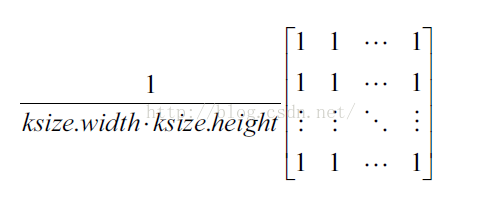3：中值滤波

4：高斯滤波

• 像均值滤波，是简单的取平均值，模板系数都是1。而图像上的像素实际上是坐标离散但是值却连续的，因为越靠近的点关系越密切，越远离的点关系越疏远。因此，加权平均更合理，距离越近的点权重越大，距离越远的点权重越小。
• 既然是依据距离来加权平均，那么很容易想到高斯函数$f\left(x\right)=\frac{1}{\sigma \sqrt{2\pi }}{e}^{\frac{-\left(x-\mu {\right)}^{2}}{2{\sigma }^{2}}}$$f(x) = \frac{1}{\sigma\sqrt{2\pi }}{e}^{\frac{-(x-\mu )^{2}}{2\sigma^2}}$，如图所示：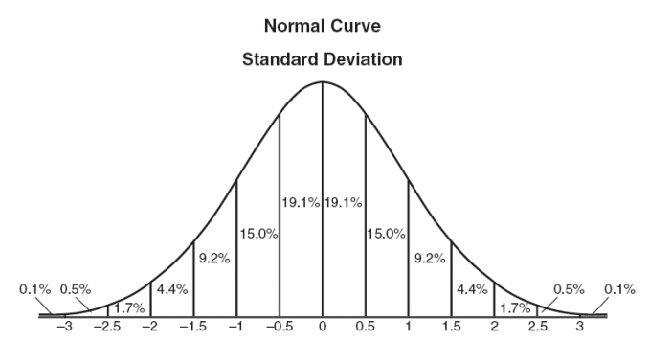从高斯函数来看，离原点距离越近，得到的权重越高，越远离原点，得到的权重越小。
上边是一维的高斯函数，当中心点为原地时，$x$$x$的均值$\mu =0$$\mu = 0$，此时$f\left(x\right)=\frac{1}{\sigma \sqrt{2\pi }}{e}^{\frac{-{x}^{2}}{2{\sigma }^{2}}}$$f(x) = \frac{1}{\sigma\sqrt{2\pi }}{e}^{\frac{-x^{2}}{2\sigma^2}}$
由于图像是二维矩阵，则采用二维高斯函数$f\left(x,y\right)=\frac{1}{2\pi {\sigma }^{2}}{e}^{\frac{-\left({x}^{2}+{y}^{2}\right)}{2{\sigma }^{2}}}$$f(x,y) = \frac{1}{{2\pi }\sigma^2}{e}^{\frac{-(x^2+y^2 )}{2\sigma^2}}$有了这个函数就可以计算滤波模板中每个点的权重了。
权重矩阵：
假定中心点是（0，0），那么距离它最近的8个点的坐标如下：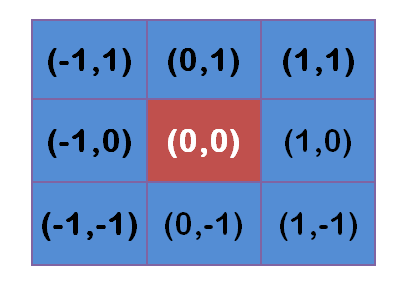更远的点以此类推。
将这模板中的坐标x,y代入到二维高斯函数中，假定$\mu =0$$\mu = 0$$\sigma$$\sigma$为1.5,则模糊半径为1的权重矩阵如下: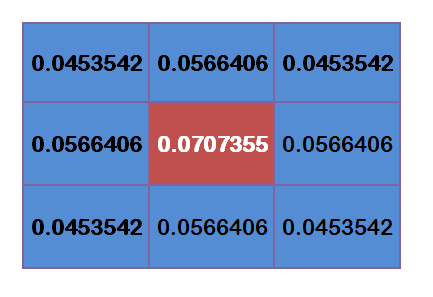这9个点的权重总和等于0.4787147，如果只计算这9个点的加权平均，还必须让它们的权重之和等于1，因此上面9个值还要分别除以0.4787147，得到最终的权重矩阵。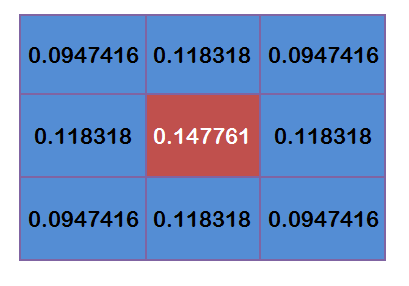计算高斯滤波的结果
假设现在3*3高斯滤波器覆盖的图像的像素灰度值为：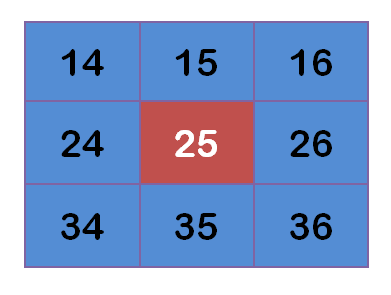每个点与上边计算得到的9个权重值相乘：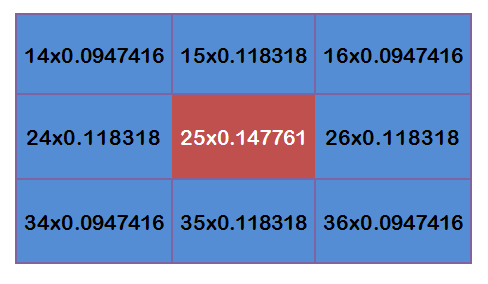得到：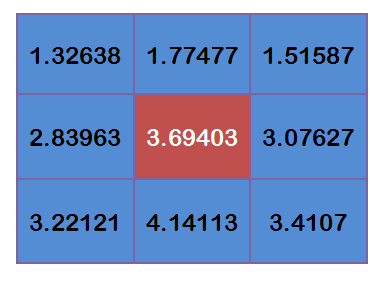将这9个值加起来，就是中心点的高斯模糊的值。

5：双边滤波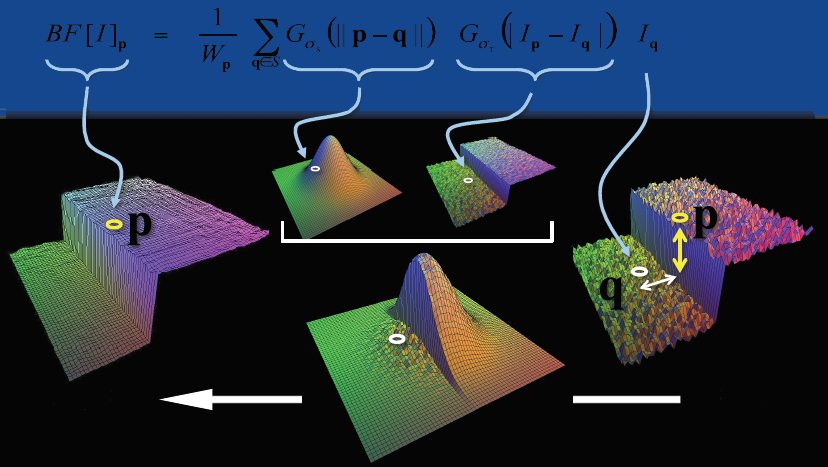## 眼底图像血管增强与分割--（1）匹配滤波算法原理及实现

2017-10-12 13:01:27 piaoxuezhong 阅读数 10832
• ###### OpenCV3.2 Java图像处理视频学习教程

OpenCV3.2 Java图像处理视频...主要内容包括opencv像素操作、滤波、边缘提取、直线与圆检测、形态学操作与分水岭、图像金子塔融合重建、多尺度模板匹配、opencv人脸检测、OpenCV跟Tomcat使用实现服务器端图像处理服务。

39课时 884分钟 4656人学习 贾志刚
免费试看

视网膜血管分割是眼科计算机辅助诊断和大规模疾病筛查系统的基础，当眼器官发生视觉疾病的时候，网膜血管的直径、颜色和弯曲程度等就会出现异常，科医生可以据此作出诊断。医疗上对血管图像进行分割常用的方法有：基于血管跟踪的方法、基于匹配滤波的方法、基于形态学处理的方法、基于形变模型的方法和基于机器学习的方法等 。本篇将介绍基于匹配滤波算法。

# 匹配滤波器(matched filter)算法

## 原理部分：

匹配滤波算法最早见于论文【1】《Detection of  Blood Vessels in Retinal Images Using Two-Dimensional Matched Filters

》中，后来又有许多针对该算法的不足进行改进的算法出现。

匹配滤波器是指经过滤波后，使得滤波器输出端的信号瞬时功率与噪声平均功率的比值（即是信噪比SNR）最大，当有用信号与噪声同时进入滤波器时，有用信号在某一瞬间出现尖峰值，而噪声信号受到抑制。

论文【1】对眼底视网膜上的血管特点进行分析，基于这些特点设计匹配滤波器，主要参考特点：血管的宽度在较小的范围内变动，血管壁的两条线是近似平行的，血管有方向，血管横切线上的灰度曲线如下，近似满足高斯分布：要理解论文中的公式推导，则需要对匹配滤波有更深层次的理解和掌握。首先，匹配滤波器也是一种在某一准则条件下的最优设计，其对应的最优的准则是输出信噪比（SNR）最大，而且前提条件是噪声信号为白噪声。论文得到的匹配滤波器的表达式为：H(f)=S*(f)，即匹配滤波器的频率响应是输入信号频率响应的共轭。从物理直观上理解：

（1）从幅频特性来看，匹配滤波器和输入信号的幅频特性一致。在信号越强的频率点，滤波器的放大倍数也越大；在信号越弱的频率点，滤波器的放大倍数越小。这就是信号处理中的“马太效应”，匹配滤波器是让有用信号尽可能通过。白噪声的功率谱在各个频率点都一样，这种情况下，有用信号尽可能通过，噪声则相反。
（2）从相频特性上看，匹配滤波器的相频特性和输入信号则相反。通过匹配滤波器后，信号的相位为0，正好能实现信号时域上的相干叠加。而噪声的相位是随机的，只能实现非相干叠加。这样在时域上保证了输出信噪比的最大。
在信号的幅频特性与相频特性中，幅频特性表征了频率特性，频特性表征了时间特性。匹配滤波器无论是从时域还是从频域，都充分保证了信号尽可能大的通过，噪声尽可能小的通过。

回到论文【1】中的描述，将血管想象成一小段一小段的平行区域的组合，设定长度为L，宽度为3σ，然后用一个高斯曲线来模拟血管横切面上的灰度曲线， 匹配滤波器如下：## 算法实现：

%% matched filter2，测试程序
clc,close all,clear all;
if length(size(img))==3
img=rgb2gray(img);
end
[g,bg]=matchedFilter2(img);

function [g,bg]=matchedFilter2(f)

f=double(f);
subplot(1,2,1);
imshow(uint8(f));
title('origin image');
% mean filter
f=medfilt2(f,[5,5]);
% f=medfilt2(f,[21 1]);
% f=medfilt2(f,[1,7]);
% 参数
os=12;  % 角度的个数
sigma=2;
tim=3;
L=9;
t=180; % 全局阈值,需要多次尝试

thetas=0:(os-1);
thetas=thetas.*(180/os);
N1=-tim*sigma:tim*sigma;
N1=-exp(-(N1.^2)/(2*sigma*sigma));
N=repmat(N1,[2*floor(L/2)+1,1]);
r2=floor(L/2);
c2=floor(tim*sigma);
[m,n]=size(f);
RNs=cell(1,os);  % rotated kernals
MFRs=cell(1,os); % filtered images
g1=f;

% matched filter
for i=1:os
theta=thetas(i);
RN=imrotate(N,theta);
%去掉多余的0行和零列
RN=RN(:,any(RN));
RN=RN(any(RN'),:);
meanN=mean2(RN);
RN=RN-meanN;
RNs{1,i}=RN;
MFRs{1,i}=imfilter(f,RN,'conv','symmetric');
end

% get the max response
g=MFRs{1,1};
for j=2:os
g=max(g,MFRs{1,j});
end
bg=g<t;

subplot(1,2,2);
imshow(bg);
title('filterd image');
end%% 测试函数，匹配滤波算法血管分割
clc,close all,clear all;
sigma=2;
yLength=10;
direction_number=12;
if length(size(img))==3
img=rgb2gray(img);
end
MF = MatchFilter(img, sigma, yLength,direction_number);
0 1 1 1 0;
0 1 1 1 0;
0 1 1 1 0;
0 0 0 0 0;];
MF = normalize(double(MF));
features = MF;
subplot(1,2,1);
imshow(img);title('origin image');
subplot(1,2,2);
imshow(features);title('filted image');## 算法优缺点：

• http://www.cnblogs.com/naniJser/archive/2012/12/09/2810551.html
• http://blog.csdn.net/chiooo/article/details/43564069
• http://blog.csdn.net/u013288466/article/details/66473284

## 眼底视网膜血管增强方法（一）匹配滤波

2017-03-26 12:18:38 u013288466 阅读数 5409
• ###### OpenCV3.2 Java图像处理视频学习教程

OpenCV3.2 Java图像处理视频...主要内容包括opencv像素操作、滤波、边缘提取、直线与圆检测、形态学操作与分水岭、图像金子塔融合重建、多尺度模板匹配、opencv人脸检测、OpenCV跟Tomcat使用实现服务器端图像处理服务。

39课时 884分钟 4656人学习 贾志刚
免费试看

# 眼底视网膜血管增强方法（一）匹配滤波

## 信号处理中的匹配滤波

r(t)=s(t)+n(t)0tTs

H(f)=kS(f)ej2πft0

## 眼底图像的匹配滤波

### 匹配原理### 匹配滤波器的构造

#### 滤波器的表达式

K(x,y)=exp(x22δ2)yL2

## 实验结果## 原程序

    sigma=2;
yLength=9;
direction_number=12;
MF = MatchFilter(img, sigma, yLength,direction_number);
MF = normalize(double(MF));
features = MF;

1. SUBHASIS CHAUDHURI, STUDENT MEMBER,”Detection of Blood Vessels in Retinal Images Using